Courses

# Mechanical Engineering - ME 2016 GATE Paper (Practice Test)

## 65 Questions MCQ Test GATE Mechanical (ME) 2022 Mock Test Series | Mechanical Engineering - ME 2016 GATE Paper (Practice Test)

Description
This mock test of Mechanical Engineering - ME 2016 GATE Paper (Practice Test) for GATE helps you for every GATE entrance exam. This contains 65 Multiple Choice Questions for GATE Mechanical Engineering - ME 2016 GATE Paper (Practice Test) (mcq) to study with solutions a complete question bank. The solved questions answers in this Mechanical Engineering - ME 2016 GATE Paper (Practice Test) quiz give you a good mix of easy questions and tough questions. GATE students definitely take this Mechanical Engineering - ME 2016 GATE Paper (Practice Test) exercise for a better result in the exam. You can find other Mechanical Engineering - ME 2016 GATE Paper (Practice Test) extra questions, long questions & short questions for GATE on EduRev as well by searching above.
QUESTION: 1

### Q. 1 – Q. 5 carry one mark each Q. Which of the following is CORRECT with respect to grammar and usage? Mount Everest is ______

Solution:

Before superlative article ‘the’ has to be used. “One of” the expression should take plural noun and so option ‘C’ and ‘D’ can’t be the answer.

QUESTION: 2

Solution:

‘lose’ is verb

QUESTION: 3

### Despite the new medicine’s ______in treating diabetes, it is not ________ widely

Solution:

‘effectiveness’ is noun and ‘prescribed’ is verb. These words are apt and befitting with the word ‘medicine’.

QUESTION: 4

Michael lives 10 km away from where I live. Ahmed lives 5 km away and Susan lives 7 km away from where I live. Arun is farther away than Ahmed but closer than Susan from where I live. From the information provided here, what is one possible distance (in km) at which I live from Arun’s place?

Solution:
QUESTION: 5

Michael lives 10 km away from where I live. Ahmed lives 5 km away and Susan lives 7km away from where I live Arun is farther away than Ahmed but closer than susan from where I live. From the information provided here, what is one possible distance (in km) at which I live from Arun’s place?

Solution: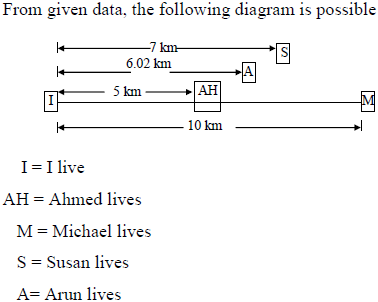QUESTION: 6

Q. 6 – Q. 10 carry two marks each.

Q. A person moving through a tuberculosis prone zone has a 50% probability of becoming infected. However only 30% of infected people develop the disease. What percentage of people moving through a tuberculosis prone zone remains infected but does not show symptoms of disease?

Solution: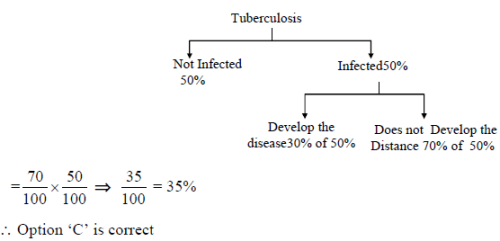QUESTION: 7

In a world filled with uncertainty he was glad to have many good friends. He had always assisted them in times of need and was confident that they would reciprocate. However, the events of the last week proved him wrong?
Which of the following inference (s) is/are logically valid and can be inferred from the above passage?
(i) His friends were always asking him to help them
(ii) He felt that when in need of help, his friends would let him down
(iii) He was sure that his friends would help him when in need
(iv) His friends did not help him last week

Solution:

The words ‘was confident that they would reciprocate’ and ‘last week proved him wrong’ lead to statements iii and iv as logically valid inferences.

QUESTION: 8

Leela is older than her cousin Pavithra. Pavithra’s brother Shiva is older than Leela. When Pavithra and Shiva are visiting Leela, all three like to play chess. Pavithra wins often than Leela does.
Which one of the following statements must be TRUE based on the above?

Solution:

From given data, the following arrangement is possible
Shiva
Leela
Pavithra
Among four alternatives, option D is TRUE.

QUESTION: 9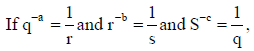the value of abc is______

Solution: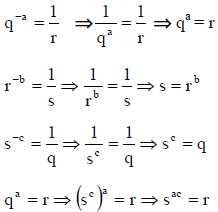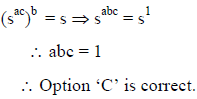QUESTION: 10

P,Q,R and S are working on a project. Q can finish the task in 25 days, working alone for 12 hours a day. R can finish the task in 50 days, working along for 12 hours per day. Q worked 12 hours a day but took sick leave in the beginning for two days. R worked 18 hours a day on all days. What is the ratio of work done by Q and R after 7 days from the start of the project?

Solution: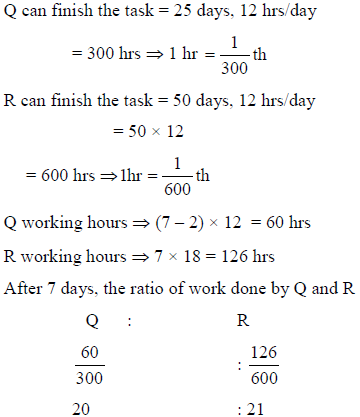QUESTION: 11

Q. 11 – Q. 35 carry one mark each:

Q. The solution to the system of equations is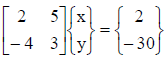Solution:

x = 6, y = –2 is the solution of equation

2x+5y = 2 and –4x + 3y = –30

QUESTION: 12

If f(t) is a function defined for all t > 0, its laplace transform F(s) is defined as

Solution:

By the definition of Laplace transform of f(t)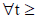, we have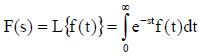QUESTION: 13

f(z) = u(x,y) + i v(x,y) is an analytic function of complex variable z = x + iy where i =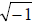. If u(x,y) = 2 xy, then v(x,y) may be expressed as

Solution:

Given u =2xy, v = ?
The Cauchy-Riemann equation
ux = vy & vx = – uy are satisfying with option (a) –x2+ y2 + constant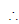V(x,y) = –x2 + y+ constant

QUESTION: 14

Consider a Poisson distribution for the tossing of a biased coin. The mean for this distribution is μ. The standard deviation for this distribution is given by

Solution:

For Poisson distribution mean = variance
given mean = μvariance = μstandard deviation =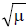*Answer can only contain numeric values
QUESTION: 15

Solve the equation x = 10 cos (x) using the Newton-Raphson method. The initial guess is x = π/4.
The value of the predicted root after the first iteration, up to second decimal, is __________.

(Important - Enter only the numerical value in the answer)

Solution: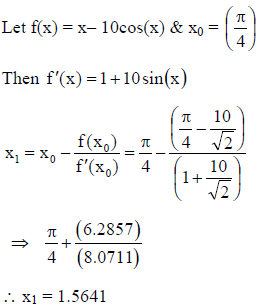*Answer can only contain numeric values
QUESTION: 16

A rigid ball of weight 100 N is suspended with the help of a string. The ball is pulled by a horizontal force F such that the string makes an angle of 30? with the vertical. The magnitude of force F (in N) is _____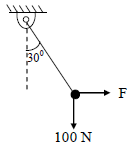Solution: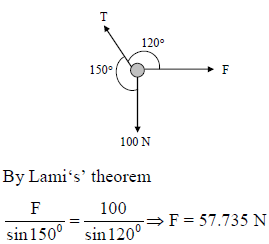QUESTION: 17

A point mass M is released from rest and slides down a spherical bowl (of radius R ) from a height H as shown in the figure below. The surface of the bowl is smooth (no friction). The velocity of the mass at the bottom of the bowl is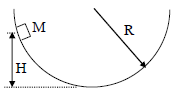Solution: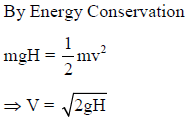QUESTION: 18

The cross sections of two hollow bars made of the same material are concentric circles as shown in the figure. It is given that r3 > r1 and r4 > r2, and that the areas of the cross-sections are the same. J1 and J2 are the torsional rigidities of the bars on the left and right, respectively. The ratio J2/J1 is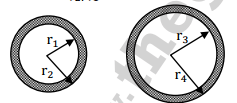Solution: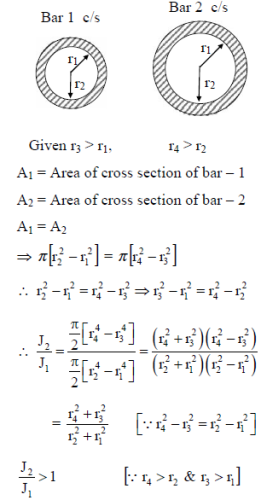QUESTION: 19

A cantilever beam having square cross-section of side a is subjected to an end load. If a is increased by 19%, the tip deflection decreases approximately by

Solution: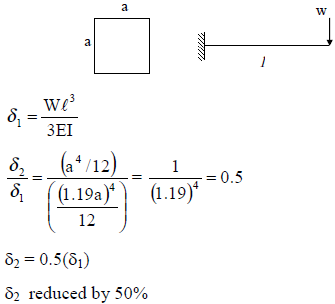*Answer can only contain numeric values
QUESTION: 20

A car is moving on a curved horizontal road of radius 100 m with a speed of 20 m/s. The rotating masses of the engine have an angular speed of 100 rad/s in clockwise direction when viewed from the front of the car. The combined moment of inertia of the rotating masses is 10 kg-m2. The magnitude of the gyroscopic moment (in N-m) is_____

Solution: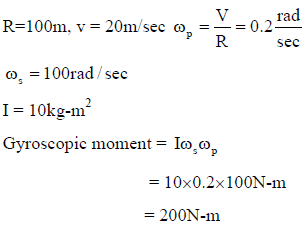*Answer can only contain numeric values
QUESTION: 21

A single degree of freedom spring mass system with viscous damping has a spring constant of 10 kN/m. The system is excited by a sinusoidal force of amplitude 100 N. If the damping factor (ratio) is 0.25, the amplitude of steady state oscillation at resonance is ________mm

Solution: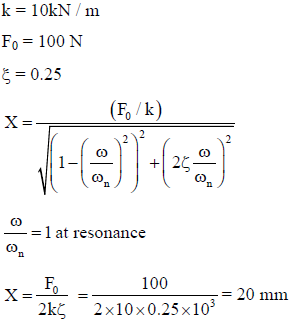QUESTION: 22

The spring constant of a helical compression spring DOES NOT depend on

Solution:
QUESTION: 23

The instantaneous stream-wise velocity of a turbulent flow is given as follows: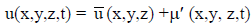The time – average of the fluctuating velocity u' (x,y, z, t)

Solution:

Time average of fluctuating velocity is zero.

QUESTION: 24

For a floating body, buoyant force acts at the

Solution:

For floating body Buoyancy force acts through the centre of buoyancy which is C.G for displaced volume.

QUESTION: 25

A plastic sleeve of outer radius ro = 1 mm covers a wire (radius r = 0.5 mm) carrying electric current. Thermal conductivity of the plastic is 0.15 W/m-K. The heat transfer coefficient on the outer surface of the sleeve exposed to air is 25 W/m2-K. Due to the addition of the plastic cover, the heat transfer from the wire to the ambient will

Solution: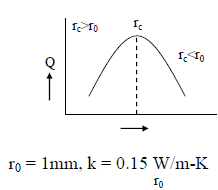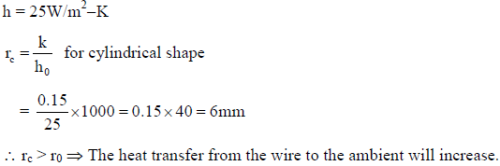QUESTION: 26

Which of the following statements are TRUE with respect to heat and work?
(i) They are boundary phenomena
(ii) They are exact differentials
(iii) They are path functions

Solution:
*Answer can only contain numeric values
QUESTION: 27

Propane (C3H8) is burned in an oxygen atmosphere with 10% deficit oxygen with respect to the stoichiometric requirement. Assuming no hydrocarbons in the products, the volume percentage of CO in the products is ____

(Important -  Enter only the numerical value in the answer)

Solution:

C3H8 + xO2 → aCO2 + bH2O
Carbon balance :
a = 3
hydrogen balance:
2b = 8 → b = 4
Oxygen balance:
2x = 2a + b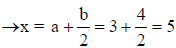For chemically correct or stoichiometric burning, no. of moles of O2 required are = 5.
As it is burnt with 10% deficient oxygen, it will generate CO.
The new equation is
C3H8 + 0.9 x 5O2 → aCO2 + bCO + cH2O
Carbon balance:
a + b = 3
Hydrogen balance:
2c = 8 → c = 4
Oxygen balance:
2a + b + c = 0.9 x 5 x 2 = 9
2a + b + c = 9
⇒ 2a + b + 4 = 9 ⇒ 2a + b = 5 … (1)
a + b = 3 … (2)
By solving (1) & (2)
a = 2 & b = 1
in the exhaust products the no. of moles of CO are 1.% by volume of CO in exhaust.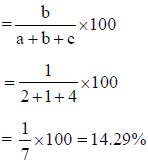*Answer can only contain numeric values
QUESTION: 28

Consider two hydraulic turbines identical specific speed and effective head at the inlet. If the speed
ratio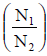of the two turbines is 2, then the respective power ratio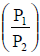_________.

Solution: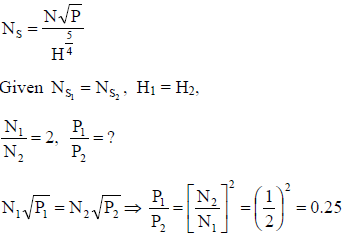QUESTION: 29

The INCORRECT statement about regeneration in vapor power cycle is that

Solution:
QUESTION: 30

The “Jominy test” is used to find

Solution:

The depth upto which the required hardness is obtained is called as hardenability and it is
determined by using jomney end quench test.

QUESTION: 31

Under optimal conditons of the process the temperatures experienced by a copper work piece in fusion welding, brazing and soldering are such that

Solution:

In welding ( Fusion welding for melting the parent material the temperature should be greater than the MP of the metal hence it is high. Whereas brazing and soldering are the nonfusion welding operations hence the temperature should be less than the MP of the metal. Brazing temp is above 4270C and soldering is below 4270C

QUESTION: 32

The part of a gating system which regulates the rate of pouring of molten metal is

Solution:

Rate of pouring of molten metal depends on the flow rate of molten metal. This depends on the choke area and it is the minimum area out of the cross sectional areas of sprue, runner and ingate.

QUESTION: 33

The non-traditional machining process that essentially requires vacuum is

Solution:

Electron beam machining is the only method carried out under vacuum, to avoid the dispersion of electrons after the magnetic deflector.

*Answer can only contain numeric values
QUESTION: 34

In an orthogonal cutting process the tool used has rake angle of zero degree. The measured cutting force and thrust force are 500 N and 250 N, respectively. The coefficient of friction between the tool and the chip is _________

Solution:

Because the rake angle is zero, F= Friction force = Fc = Cutting force = 500N, N = Normal to friction force = Ft = Thrust force = 250N,

Coefficient of friction = F/N = 250 / 500 = 0.5

QUESTION: 35

Match the following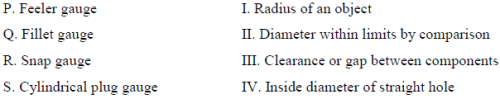Solution:

Feeler gauge is used for checking the clearance or gap between the parts, radius is checked by fillet gauge, limits of diameter of shaft is checked by snap gauge and plug gauge is used for checking the diameter of hole.

*Answer can only contain numeric values
QUESTION: 36

Q.36 to 65 (Two marks Questions)

Q. Consider the function f(x) = 2x3 -3x2 in the domain [-1, 2]. The global minimum of f(x) is ____

(Important - Enter only the numerical value in the answer)

Solution: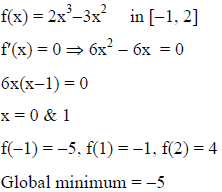*Answer can only contain numeric values
QUESTION: 37

If y = f(x) satisfies the boundary value problem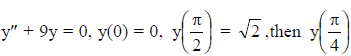is ________.

Solution: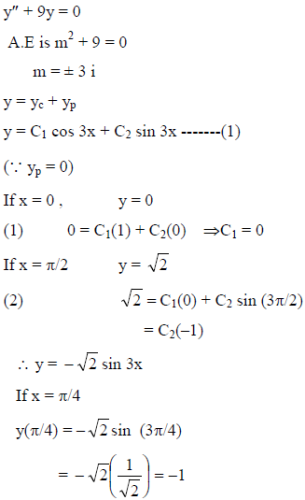QUESTION: 38

The value of the integral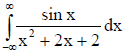evaluated using contour integration and the residue theorem is

Solution: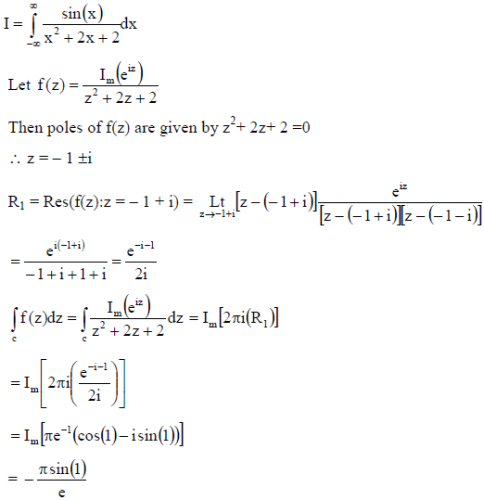*Answer can only contain numeric values
QUESTION: 39

Gauss-Seidel method is used to solve the following equations (as per the given order).
x1 + 2x2 + 3x3 = 5
2x1 + 3x2 + x3 = 1
3x1 + 2x2 + x3 = 3
Assuming initial guess as x1 = x2 = x3 = 0, the value of x3 after the first iteration is ________

(Important - Enter only the numerical value in the answer)

Solution: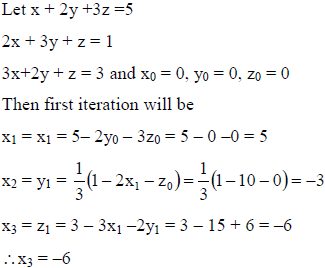*Answer can only contain numeric values
QUESTION: 40

A block of mass m rests on an inclined plane and is attached by a string to the wall as shown in the figure. The coefficient of static friction between the plane and the block is 0.25. The string can withstand a maximum force of 20 N. The maximum value of the mass (m) for which the string will not break and the block will be in static equilibrium is ___kg.
Take cosθ = 0.8 and sin θ = 0.6.
Acceleraiton due to gravity g = 10 m/s2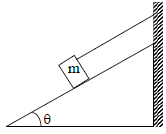Solution: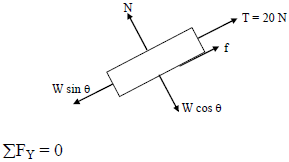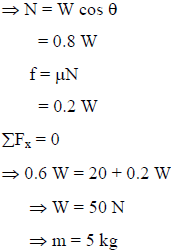QUESTION: 41

A two – member truss PQR is supporting a load W. The axial forces in members PQ and QR are respectively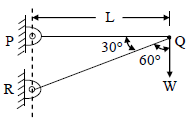Solution: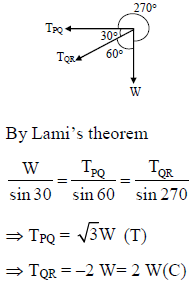*Answer can only contain numeric values
QUESTION: 42

A horizontal bar with a constant cross-section is subjected to loading as shown in the figure. The Young’s moduli for the sections AB and BC are 3E and E, respectively.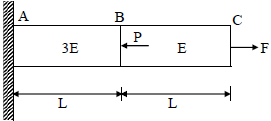For the deflection at C to be zero, the ratio P/F is ____

(Important - Enter only the numerical value in the answer)

Solution: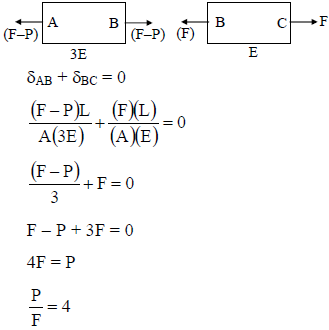*Answer can only contain numeric values
QUESTION: 43

The figure shows cross-section of a beam subjected to bending. The area moment of inertia (in mm4) of this cross-section about its base is ________

(Important -  Enter only the numerical value in the answer)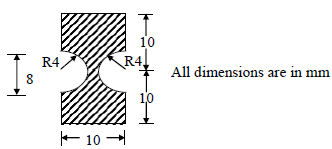Solution: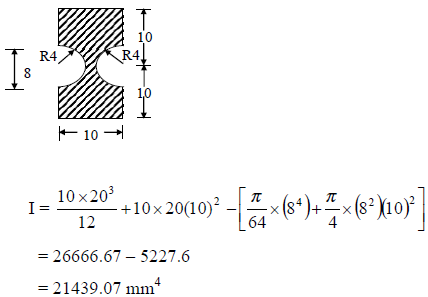*Answer can only contain numeric values
QUESTION: 44

A simply-supported beam of length 3L is subjected to the loading shown in the figure.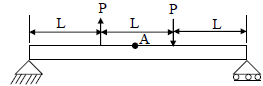It is given that P = 1 N, L = 1 m and Young’s modulus E = 200 GPa. The cross-section is a square with dimension 100 mm. The bending stress (in Pa) at the point A located at the top surface of the beam at a distance of 1.5L from the left end is (Indicate compressive stress by a negative sign and tensile stress by a positive sign).

(Important - Enter only the numerical value in the answer)

Solution: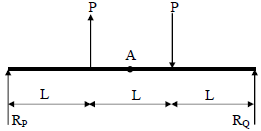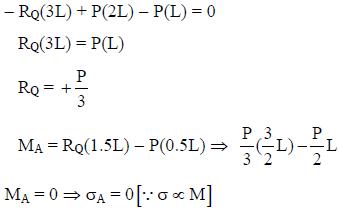*Answer can only contain numeric values
QUESTION: 45

A slider crank mechanism with crank radius 200 mm and connecting rod length 800 mm is shown. The crank is rotating at 600 rpm in the counterclockwise direction. In the configuration shown, the crank makes an angle of 90o with the sliding direction of the slider, and a force of 5 kN is acting on the slider. Neglecting the inertia forces, the turning moment on the crank (in kN-m) is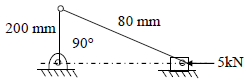(Important - Enter only the numerical value in the answer)

Solution: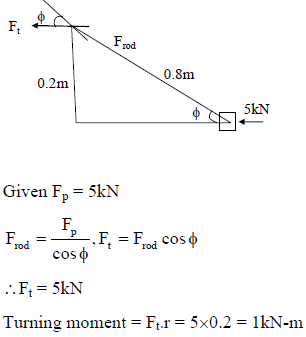QUESTION: 46

In the gear train shown, gear 3 is carried on arm 5. Gear 3 meshes with gear 2 and gear 4. The number of teeth on gear 2,3, and 4 are 60, 20, and 100, respectively. If gear 2 is fixed and gear 4 rotates with an angular velocity of 100 rpm in the counterclockwise direction, the angular speed of arm 5 (in rpm) is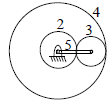Solution: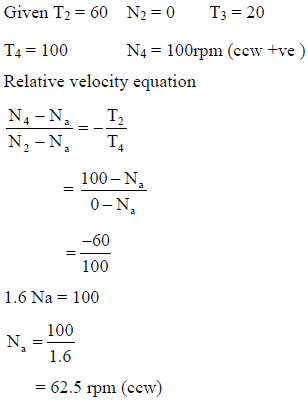QUESTION: 47

A solid disc with radius a is connected to a spring at a point d above center of the disc. The other end of the spring is fixed to the vertical wall. The disc is free to roll without slipping on the ground. The mass of the disc is M and the spring constant is K. The polar moment of inertia for the disc about its centere is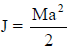The natural frequency of this system in rad/s is given by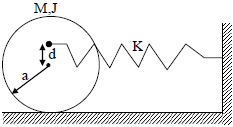Solution: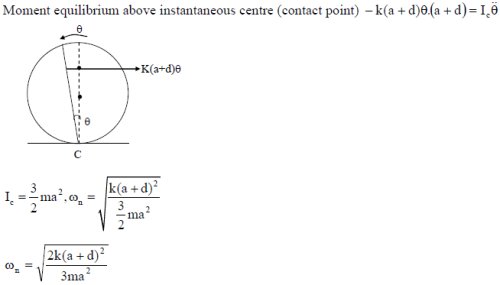QUESTION: 48

The principal stresses at a point inside a solid object are σ1 = 100 MPa, σ2 = 100 MPa and σ3 = 0 MPa. The yield strength of the material is 200 MPa. The factor of safety calculated using Tresca (maximum shear stress) theory is nT and the factor of safety calculated using von Mises (maximum distortional energy) theory is nv. Which one of the following relations is TRUE?

Solution: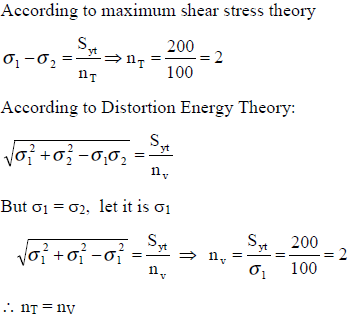*Answer can only contain numeric values
QUESTION: 49

An inverted U-tube manometer is used to measure the pressure difference between two pipes A and B, as shown in the figure. Pipe A is carrying oil (Specific gravity = 0.8) and Pipe B is carrying water. The densities of air and water are 1.16 kg/m and 1000 kg/m3, respectively. The pressure difference between pipes A and B is _______kPa.
Acceleration due to gravity g = 10 m/s2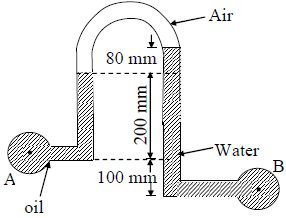(Important - Enter only the numerical value in the answer)

Solution: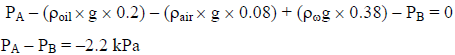*Answer can only contain numeric values
QUESTION: 50

Oil (kinematic viscosity, νoil = 1.0 x 10-5 m2/s) flows through a pipe of 0.5 m diameter with velocity of 10 m/s. Water (Kinematic viscosity, νw = 0.89 x 10-6 m2/s) is flowing through a model pipe of diameter 20 mm. For satisfying the dynamic similarity, the velocity of water (in m/s) is ____

(Important - Enter only the numerical value in the answer)

Solution: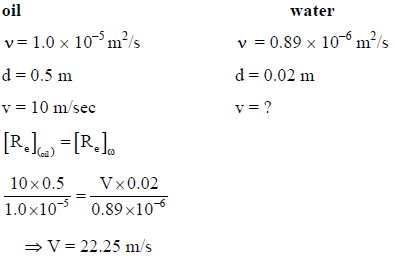*Answer can only contain numeric values
QUESTION: 51

A steady laminar boundary layer is formed over a flat plate as shown in the figure. The free stream velocity of the fluid is Uo . The velocity profile at the inlet a-b is uniform, while that at a downstream location c-d is given by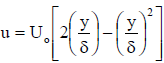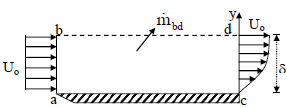The ratio of the mass flow rate, bd, mbd leaving through the horizontal section b-d to that entering through the vertical section a-b is

(Important - Enter only the numerical value in the answer)

Solution: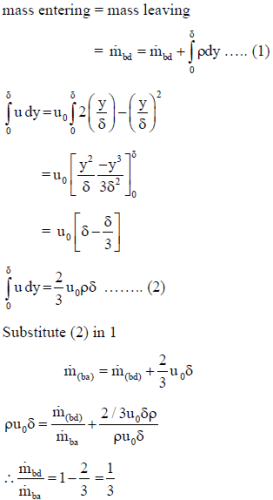*Answer can only contain numeric values
QUESTION: 52

A steel ball of 10 mm diameter at 1000 K is required to be cooled to 350 K by immersing it in a water environment at 300 K. The convective heat transfer coefficient is 1000 W/m2-K. Thermal conductivity of steel is 40 W/m-K.The time constant for the cooling process τ is 16s. The time required (in s) to reach the final temperature is________

(Important - Enter only the numerical value in the answer)

Solution: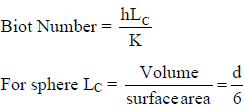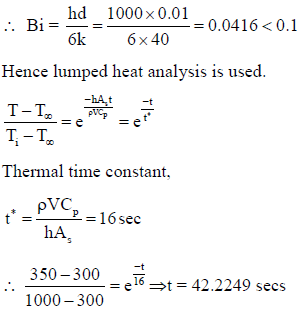*Answer can only contain numeric values
QUESTION: 53

An infinitely long furnace of 0.5 m x 0.4 m cross-section is shown in the figure below. Consider all surfaces of the furnace to be black. The top and bottom walls are maintained at temperature T1 = T3 = 927oC while the side walls are at temperature T2 = T4 = 527oC. The view factor, F1-2 is 0.26. The net radiation heat loss or gain on side 1 is _____ W/m.

Stefan-Boltzman constant = 5.67 x 10-8 W/m2-K4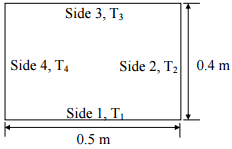(Important - Enter only the numerical value in the answer)

Solution: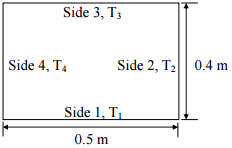T1 = 927oC = 1200K,

T2 = 527oC = 800K

F12 = F14 = 0.26

F11 + F12 + F13 + F14 = 1

F13 = 0.48

Q = Q12 + Q13 + Q14

Q13 = 0 since the temperatures are same

Q = Q12 + Q14 = 2×σb×A×F12(T14 - T24)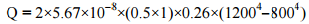= 24530.688 Watt

*Answer can only contain numeric values
QUESTION: 54

A fluid (Prandtl number, Pr = 1) at 500 K flows over a flat plate of 1.5 m length, maintained at 300 K. The velocity of the fluid is 10 m/s. Asuming kinematic viscosity, v = 30 x 10-6 m2/s, the thermal boundary layer thickness (in mm) at 0.5 m from the leading edge is ____

(Important - Enter only the numerical value in the answer)

Solution: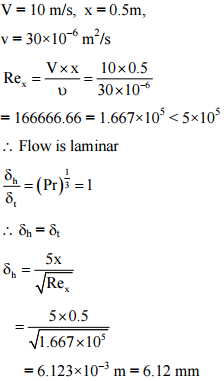*Answer can only contain numeric values
QUESTION: 55

For water at 25oC, dps/dTs = 0.189 kPa/K (ps is the saturation pressure in kPa and Ts is the saturation temperature in K) and the specific volume of dry saturated vapour is 43.38 m3/kg. Assume that the specific volume of liquid is negligible in comparson with that of vapour. Using the Clausius-Clapeyron equation, an estimate of the enthalpy of evaporation of water at 25oC (in kJ/kg) is ___

(Important - Enter only the numerical value in the answer)

Solution: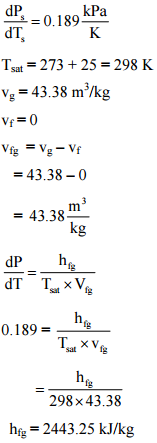*Answer can only contain numeric values
QUESTION: 56

An ideal gas undergoes a reversible process in which the pressure varies linearly with volume. The conditions at the start (subscript 1) and at the end (subscript 2) of the process with usual notation are: p1 = 100 kPa, V1 = 0.2 mand p2 = 200 kPa, V2 = 0.1 m3 and the gas constant, R = 0.275 kJ/kg-K. The magnitude of the work required for the process (in kJ) is______

(Important - Enter only the numerical value in the answer)

Solution: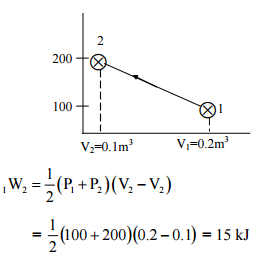*Answer can only contain numeric values
QUESTION: 57

In a steam power plant operating on an ideal Rankine cycle, superheated steam enters the turbine at 3 MPa and 350oC. The condenser pressure is 75 kPa. The thermal efficiency of the cycle is _______ percent.

Given data:

For saturated liquid, at P = 75 kPa,

hf = 384.39 kJ/kg, vf = 0.001037 m3/kg,

sf = 1.213 kJ/kg-K

At 75 kPa, hfg = 2278.6 kJ/kg,

sfg = 6.2434 kJ/kg-K

At P = 3 MPa and T = 350o C (Superheated steam), h = 3115.3 kJ/kg,

s = 6.7428 kJ/kg-K

(Important - Enter only the numerical value in the answer)

Solution: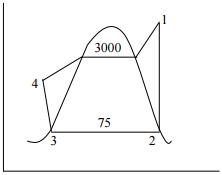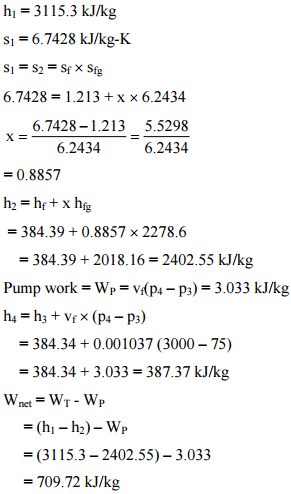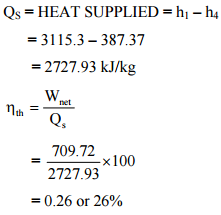*Answer can only contain numeric values
QUESTION: 58

A hypothetical engineering stress-strain curve shown in the figure has three straight lines PQ, QR, RS with coordinates P(0,0), Q (0.2,100), R(0.6, 140) and S(0.8, 130). ‘Q’ is the yield point, 'R' is the UTS point and 'S' the fracture point.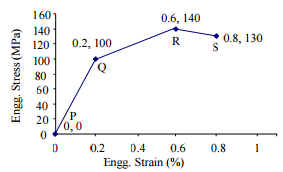The toughness of the material (in MJ/m3) is ____

(Important - Enter only the numerical value in the answer)

Solution: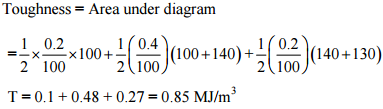*Answer can only contain numeric values
QUESTION: 59

Heat is removed from a molten metal of mass 2 kg at a constant rate of 10 kW till it is completely solidified. The cooling curve is shown in the figure.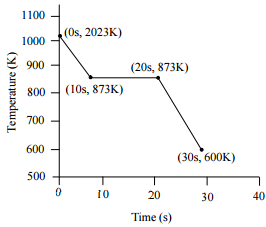Assuming uniform temperature throughout the volume of the metal during solidification, the latent heat of fusion of the metal (in kJ/kg) is ____

Solution:

m = 2 kg, Q = 10 kW

time taken for removing latent heat = 20 – 10 = 10 sec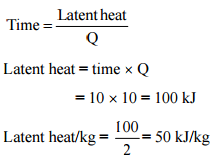QUESTION: 60

The too life equation for HSS tool is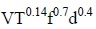= Constant. The tool life (T) of 30 min is obtained using the following cutting conditions = 45 m/min, f = 0.35 mm, d = 2.0 mm. If speed (V), feed (f) and depth of cut (D) are increased individually by 25%, the tool life (in min) is

Solution: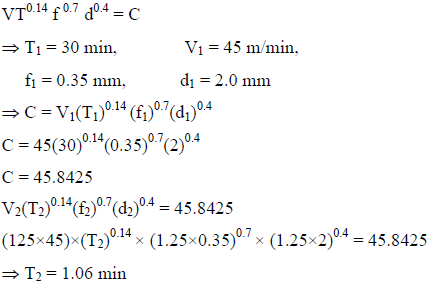QUESTION: 61

A cylindrical job with diameter of 200 mm and height of 100 mm is to be cast using modulus method of riser design. Assume that the bottom surface of cylindrical riser does not contribute as cooling surface. If the diameter of the riser is equal to its height, then the height of the riser (in mm) is

Solution: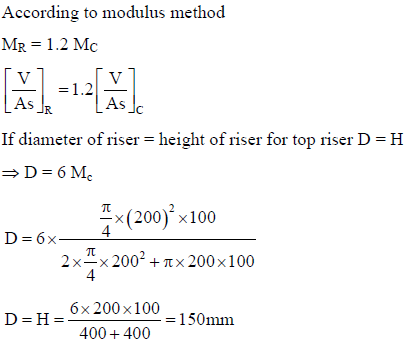*Answer can only contain numeric values
QUESTION: 62

A 300 mm thick slab is being cold rolled using roll of 600 mm diameter. If the coefficient of friction is 0.08, the maximum possible reduction (in mm) is ______

(Important - Enter only the numerical value in the answer)

Solution:

Maximum possible reduction =

ΔH/ pass = μ2R = 0.082 x 300 = 1.92 mm

QUESTION: 63

The figure below represents a triangle PQR with initial coordinates of the vertices as P(1,3), Q (4,5) and R(5,3.5). The triangle is rotated in the X-Y plane about the vertex P by angle θ in clockwise direction. If sin θ = 0.6 and cos θ = 0.8, the new coordinates of the vertex Q are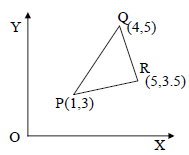Solution: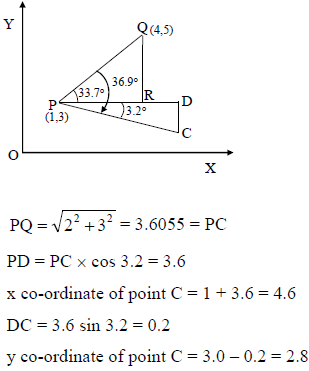*Answer can only contain numeric values
QUESTION: 64

The annual demand for an item is 10,000 units. The unit cost is Rs. 100 and inventory carrying charges are 14.4% of the unit cost per annum. The cost of one procurement is Rs. 2000. The time between two consecutive orders to meet the above demand is _____ month (s)

(Important - Enter only the numerical value in the answer)

Solution: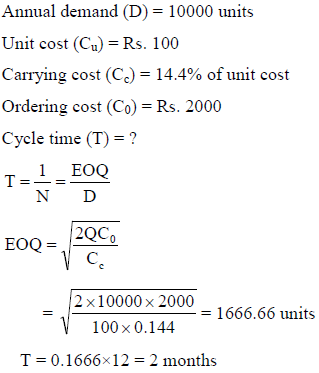QUESTION: 65

Maximize Z = 15X1 + 20X2
Subject to
12X1 + 4X2 > 36
12X1 - 6X2 < 24
X1, X2 > 0
The above linear programming problem has

Solution:

Max Z = 15x1 + 20x2
Subjected to
12x1 + 4x2 ≥ 36
12x1 - 6x2 ≤ 24
x1, x2 ≥ 0 ,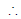unbounded solution.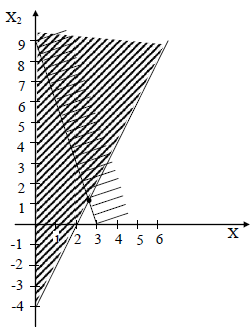Track your progress, build streaks, highlight & save important lessons and more!

### Similar Content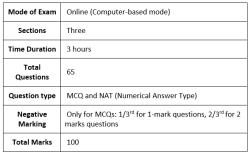### Related tests